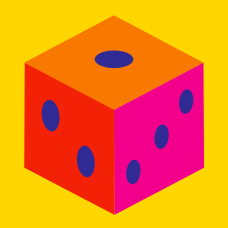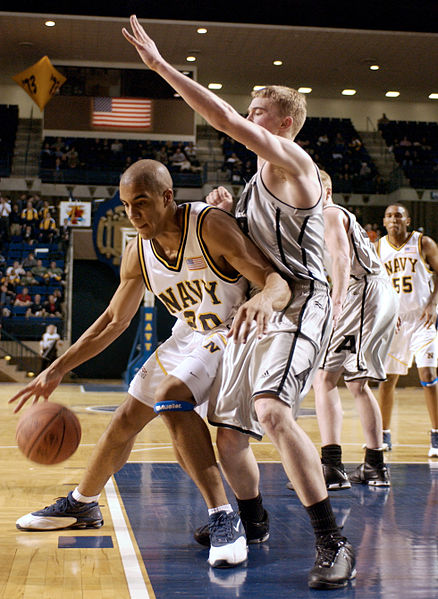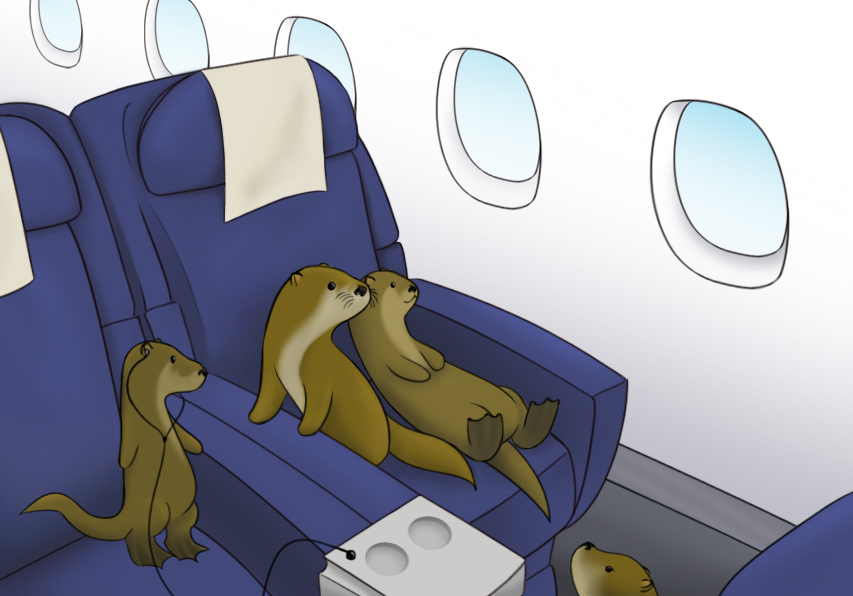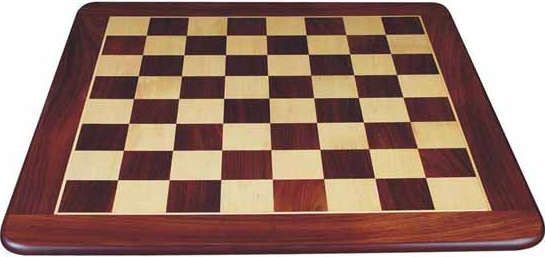Quantitative Finance

# Probability Theory: Level 3 ChallengesHow many ways are there to split 10 people into 2 teams so that they can play 5 on 5 basketball?There are 100 people in line to board a plane with 100 seats. The first person has lost his boarding pass, so he takes a random seat.

Everyone that follows takes their assigned seat if it's available, but otherwise takes a random unoccupied seat. What is the probability the last passenger ends up in his/her assigned seat, as a decimal?

Suppose there are 20 coins in a bag, 14 of which are fair, (i.e., one side shows heads and the other tails), 4 of which show heads on both sides and 2 of which show tails on both sides. (The coins are otherwise identical to the touch.)

A coin is chosen from the bag at random, and one of its sides, again chosen at random, is observed. Given that the side observed shows heads, what is the probability that the other side of this coin also shows heads?

If the probability is $$\dfrac{a}{b},$$ where $$a$$ and $$b$$ are positive coprime integers, then find $$a + b.$$If three $$1\times1$$ squares are randomly chosen from a standard $$8\times8$$ chessboard, the probability that they all lie on the same diagonal is $$\frac ab$$ for coprime positive integers, find the value of $$a+b$$.

The product of the digits in the number 126, is $$1 \times 2 \times 6 = 12$$. How many other three digit numbers have a product that is equal to 12?

×

Problem Loading...

Note Loading...

Set Loading...